Courses

# Test: Stereoisomerism Isomerism

## 21 Questions MCQ Test Chemistry for JEE | Test: Stereoisomerism Isomerism

Description
This mock test of Test: Stereoisomerism Isomerism for JEE helps you for every JEE entrance exam. This contains 21 Multiple Choice Questions for JEE Test: Stereoisomerism Isomerism (mcq) to study with solutions a complete question bank. The solved questions answers in this Test: Stereoisomerism Isomerism quiz give you a good mix of easy questions and tough questions. JEE students definitely take this Test: Stereoisomerism Isomerism exercise for a better result in the exam. You can find other Test: Stereoisomerism Isomerism extra questions, long questions & short questions for JEE on EduRev as well by searching above.
QUESTION: 1

Solution:
QUESTION: 2

Solution:
QUESTION: 3

### How many stereoisomers exist for the compound 4-(1- propenyl) cyclohexane ?

Solution:

The correct answer is option C
1,4-Dimethylcyclohexane consists of two diastereomeric stereoisomers, although, according to the n2 rule, four stereoisomers should be expected due to the fact that 1,4-dimethylcyclohexane contains two asymmetric carbons.

QUESTION: 4

what is incorrect regarding cis -1, 3-dibromo - trans-2,4-dichlorocyclobutane ?

Solution:
QUESTION: 5

consider the following zonolysis reaction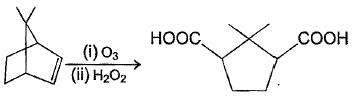The correct statement about the above product formed is

Solution:
QUESTION: 6

A hydrocarbon with molecular formula C6H8 is chiral but upon catalytic hydrogenation gives achiral hydrocarbon of molar mass 86. Which of the following could be the starting compound?

Solution: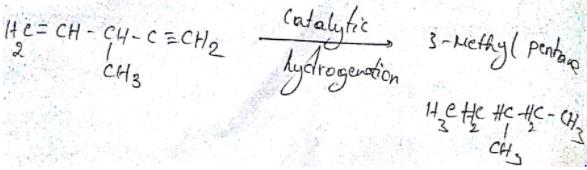*Answer can only contain numeric values
QUESTION: 7

Direction (Q. Nos. 7 and 14) This section contains 8 questions. when worked out will result in an integer from 0 to 9 (both inclusive)

Q.
How many stereoisomers exist in the compound 1-methyl-3-(1-propenyl) cyclohexane ?

Solution: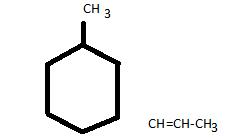Chiral Carbon
Carbon no.1 is chiral carbon so H Show 2 optical isomers
Carbon no.3 is also chiral carbon
Hence, shows two optical isomers
Carbon 1 & carbon 3 shows G.I. due to 1 stereocenter
Propenyl shows 2 G.I.
Total 8 isomers are shown here.

*Answer can only contain numeric values
QUESTION: 8

If 3-ethylpentane is subjected to free radical chlorination, how many different monochlorination product would be produced?

Solution:
*Answer can only contain numeric values
QUESTION: 9

How many chiral carbons are present in the compound shown below ?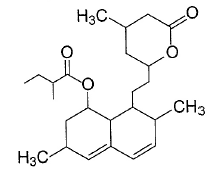Solution: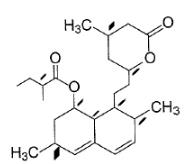All the highlighted position are chiral centres.

*Answer can only contain numeric values
QUESTION: 10

How many pairs of diastereomers can be made for the compound shown below ?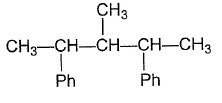Solution:
*Answer can only contain numeric values
QUESTION: 11

In principle, how many different monocarboxylic isomers, on decarboxylation with soda lime, can give the same 3-methyl pentane?

Solution:
*Answer can only contain numeric values
QUESTION: 12

How many isomers are possible for C3H2Cl2 ?

Solution:

There are 9 isomers of C3H2Cl2.
They are:-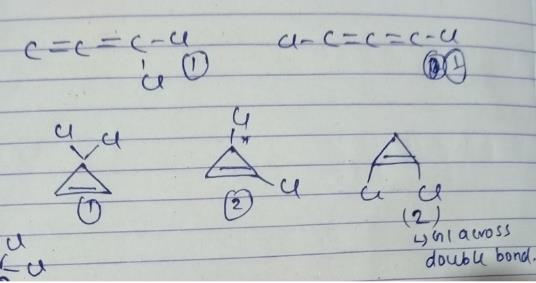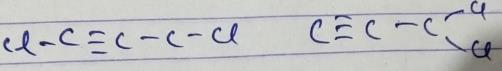*Answer can only contain numeric values
QUESTION: 13

How many stereoisomers are possible for 5-chloro-2,3hexadiene ?

Solution:

2-chloro-4-methyl hex-2-ene has a double bond, so has two geometrical isomers.
It has one asymmetric carbon atom.
So, it has 2 optical isomers
So, total isomers =2+2=4

*Answer can only contain numeric values
QUESTION: 14

How many different monoenols exist for 4-methyl-2,5-heptanedione ?

Solution:

Because it can exist in the keto form and a indol form and it was isomerization taking place during
chromatography which causes the G c peak to  diffuse

QUESTION: 15

Direction (Q. Nos. 15-18) This sectionis based on statement I and Statement II. Select the correct answer from the code given below
Q.
Statement I A racemic mixture is optically inactive.
Statement II Racemic mixture contain a pair of enantiomers.

Solution:

The correct answer is Option A.

Racemic mixture is an equimolecular mixture of enantiomeric pairs. The rotation caused by the molecules of one enantiomer is cancelled by the rotation caused by the molecules of another enantiomer.

QUESTION: 16

Q.

Statement I Cis-1,4-dichlorocyclobutane is optically inactive.
Statement II It possesses plane of symmetry.

Solution:
QUESTION: 17

Statement II Enantiomers of 2,3-pentadiene are non-interconvertible due to very large rotational barrier.

Solution:
QUESTION: 18

Q.

Statement I : The Compound shown below is optically inactive.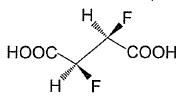Statement II Compound shown above possesses axis of rotation.

Solution:
QUESTION: 19

Q.

Statement I Decreasing length of sample tube, while keeping everything else intact, decreases the magnitude of optical rotation.
Statement II Decreasing length of sample tube decreases the contribution of sample tube material to the total optical rotation.

Solution:
QUESTION: 20

Direction (Q. Nos. 20-21) Choice the correct combination of elements and column I and coloumn II  are given as option (a), (b), (c) and (d), out of which ONE option is correct.

Q.

Consider the molecules in Column I and match them with their stereochemical properties from Column II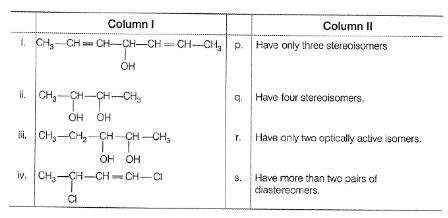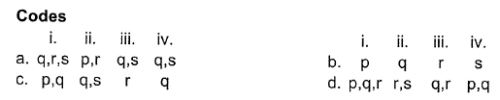Solution:
QUESTION: 21

Match the stereochemical terms in column I with their description in column II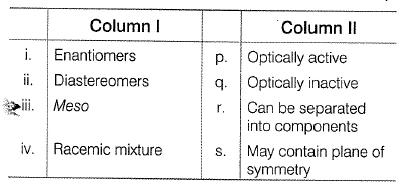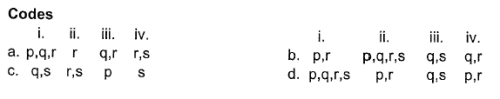Solution: